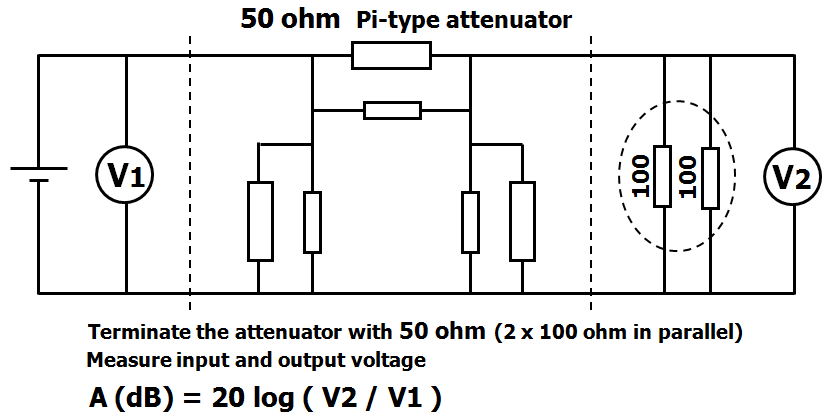### Measurement of attenuation

After building an attenuator, the attenuation can be easily and accurately measured with a DC voltage.  Voltages can measured accurately with a digital voltmeter.

Termination
To get the wanted attenuation, the attenuator must always be terminated with a 50 ohms.
During the measurement, the attenuator must be terminated with an 50 ohm resistor, that is connected to the output.
Two resistors of 100 ohm in parallel form an accurate 50 ohm resistor.
After the measurement, this 50 ohm resistor, must be removed.PA1B    Simple measurement of attenuation

Input voltage
An attenuator built with resistors of 1/4 watt, can dissipate slightly more 250 mW.
So for an attenuator with 1/4 watt resistors the voltage may not exceed 3.5 V

Measurement
Apply a voltage to the input.
Measure the voltage at the input (V1) with the digital voltmeter.
Then measure the voltage at the output (V2) with a (or the samedigital voltmeter

Calculation of the attenuation
A (in dB) = 20 * log (V2 /V1)

Example calculation
V1 is adjusted to to 3.2 V.
V2 is measured as 0.99 V
A (dB) = 20 * log (V2 / V1) = 20 * log ( 0.99 / 3.2)
A (dB) = 20 * log (0.309) = 20 * 0.5095 = -10.19 dB   (Thus an attenuation of 10,2 dB)
These values were measured at a 10 dB attenuator.